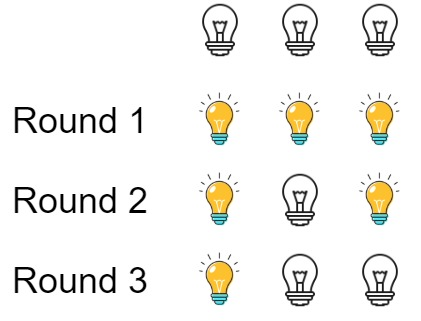### LeetCode• ㊗️
• 大家
• offer
• 多多！

## Problem

There are n bulbs that are initially off. You first turn on all the bulbs, then you turn off every second bulb.

On the third round, you toggle every third bulb (turning on if it’s off or turning off if it’s on). For the ith round, you toggle every i bulb. For the nth round, you only toggle the last bulb.

Return the number of bulbs that are on after n rounds.

Example 1:Input: n = 3
Output: 1
Explanation: At first, the three bulbs are [off, off, off].
After the first round, the three bulbs are [on, on, on].
After the second round, the three bulbs are [on, off, on].
After the third round, the three bulbs are [on, off, off].
So you should return 1 because there is only one bulb is on.


Example 2:

Input: n = 0
Output: 0


Example 3:

Input: n = 1
Output: 1


## Code

(int)Math.sqrt(n) 这样可以找出有0-n之间有多少个完全平方数 比如n=37有1,2,3,4,5,6这几个完全平方数，所以结果等于6

class Solution {
public int bulbSwitch(int n) {
return (int)Math.sqrt(n);
}
}

class Solution:
def bulbSwitch(self, n: int) -> int:
return int(math.sqrt(n))